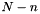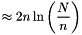pyclustering.utils.sampling Namespace Reference

Module provides various random sampling algorithms. More...

## Functions

def reservoir_r (data, n)
Performs data sampling using Reservoir Algorithm R. More...

def reservoir_x (data, n)
Performs data sampling using Reservoir Algorithm X. More...

## Detailed Description

Module provides various random sampling algorithms.

Date
2014-2020

## ◆ reservoir_r()

 def pyclustering.utils.sampling.reservoir_r ( data, n )

Performs data sampling using Reservoir Algorithm R.

Algorithm complexity O(n). Implementation is based on paper . Average number of uniform random variates:.

Parameters
 [in] data (list): Input data for sampling. [in] n (uint): Size of sample that should be extracted from 'data'.
Returns
(list) Sample with size 'n' from 'data'.

Generate random samples with 5 elements and with 3 elements using Reservoir Algorithm R:

data = [1, 2, 3, 4, 5, 6, 7, 8, 9, 10, 11, 12, 13, 14, 15, 16, 17, 18, 19, 20]
sample = reservoir_r(data, 5) # generate sample with 5 elements for 'data'.
print(sample)
sample = reservoir_r(data, 3) # generate sample with 3 elements for 'data'.
print(sample)

Output example for the code above:

[20, 7, 17, 12, 11]
[12, 2, 10]

Definition at line 30 of file sampling.py.

## ◆ reservoir_x()

 def pyclustering.utils.sampling.reservoir_x ( data, n )

Performs data sampling using Reservoir Algorithm X.

Algorithm complexity O(n). Implementation is based on paper . Average number of uniform random variates:Parameters
 [in] data (list): Input data for sampling. [in] n (uint): Size of sample that should be extracted from 'data'.
Returns
(list) Sample with size 'n' from 'data'.

Generate random sample with 5 elements using Reservoir Algorithm X:

data = [0, 1, 2, 3, 4, 5, 6, 7, 8, 9, 10, 11, 12, 13, 14, 15, 16, 17, 18, 19, 20]
sample = reservoir_x(data, 10) # generate sample with 5 elements for 'data'.
print(sample)

Output example for the code above:

[0, 20, 2, 16, 13, 15, 19, 18, 10, 9]

Definition at line 72 of file sampling.py.